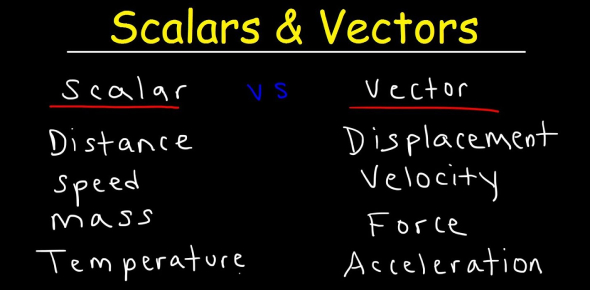# Quiz: Scalar And Vector Quantity In Physics

19 Questions | Total Attempts: 12430SettingsScalar and Vector are just two of the many quantities used in physics. Scalar is a quantity that is totally described by magnitude or size, whereas, a vector quantity is specified by both magnitudes as well as direction. So, here in this quiz, we are going to ask you nineteen questions about the same, read them carefully and answer correctly.

• 1.
Which of the following is a physical quantity that has a magnitude but no direction?
• A.

Vector

• B.

Frame of reference

• C.

Resultant

• D.

Scalar

• 2.
Multiplying or dividing vectors by scalars results in:
• A.

Vectors if multiplied or scalars if divided

• B.

Scalars if multiplied scalars

• C.

Scalars

• D.

Vectors

• 3.
Identify the following quantities as scalar or vector: the mass of an object, the number of leaves on a tree, wind velocity.
• A.

Vector, scalar, scalar

• B.

Vector, scalar, vector

• C.

Scalar, scalar, vector

• D.

Scalar, vector, vector

• 4.
Which of the following is an example of a vector quantity?
• A.

Temperature

• B.

Velocity

• C.

Volume

• D.

Mass

• 5.
Which of the following is a physical quantity that has both magnitude and direction?
• A.

Vector

• B.

Scalar

• C.

Frame of reference

• D.

Resultant

• 6.
Identify the following quantities as scalar or vector: the speed of a snail, the time it takes to run a mile, the free-fall acceleration.
• A.

Vector, scalar, scalar

• B.

Scalar, scalar, vector

• C.

Vector, scalar, vector

• D.

Scalar, vector, vector

• 7.
An ant on a picnic table travels 3.0*10^1 cm eastward, then 25 cm northward, and finally 15 cm westward. What is the magnitude  of the ant's displacement relative to its original position?
• A.

29 cm

• B.

70 cm

• C.

57 cm

• D.

52 cm

• 8.
For the winter, a duck flies 10.0 m/s due south against a gust of wind with a speed of 2.5 m/s. What is the resultant velocity of the duck?
• A.

-7.5 m/s south

• B.

12.5 m/s south

• C.

7.5 m/s south

• D.

-12.5 m/s south

• 9.
In a coordinate system, a vector is oriented at an angle with respect to the x-axis. The y-component of the vector equals the vector's magnitude multiplied by which trigonometric function?
• A.

Tan angle

• B.

Cos angle

• C.

Cot angle

• D.

Sin angle

• 10.
In a coordinate system, a vector is oriented at an angle with respect to the x-axis. The x-component of the vector equals the vector's magnitude multiplied by which trigonometric function?
• A.

Tan angle

• B.

Cos angle

• C.

Cot angle

• D.

Sin angle

• 11.
Which of the following is the motion of objects moving in two dimensions under the influence of gravity?
• A.

Vertical velocity

• B.

Horizontal velocity

• C.

Directrix

• D.

Projectile motion

• 12.
Which of the following does not exhibit  parabolic motion?
• A.

A baseball thrown to home plate

• B.

A frog jumping from land into water

• C.

A flat piece of paper released from a window

• D.

A basketball thrown to a hoop

• 13.
What is the path of a projectile (in the absence of friction)?
• A.

A wavy line

• B.

Projectiles do not follow a predictable path.

• C.

A hyperbola

• D.

A parabola

• 14.
Which of the following is not an example of projectile motion?
• A.

A volleyball served over a net

• B.

A baseball hit by a bat

• C.

A hot-air balloon drifting toward Earth

• D.

A long jumper in action

• 15.
Which of the following exhibits parabolic motion?
• A.

A space shuttle orbiting Earth

• B.

A leaf falling from a tree

• C.

A stone thrown into a lake

• D.

A train moving along a flat track

• 16.
Which of the following is an example of projectile motion?
• A.

An aluminum can dropped straight down into the recycling bin

• B.

A thrown baseball

• C.

A spaece shuttle being launched

• D.

A jet lifting off a runway

• 17.
Which of the following is a coordinate system for specifying the precise location of objects in space?
• A.

Diagram

• B.

X-axis

• C.

Frame of reference

• D.

Y-axis

• 18.
A piece of chalk is dropped by a teacher walking at a speed of 1.5 m/s. From the teacher's perspective, the chalk appears fall
• A.

Straight down

• B.

Straight backward

• C.

Straight down and forward

• D.

Straight down and backward

• 19.
A passenger on a bus moving east sees a man standing on a curb. From the passenger's perspective, the man appears to
• A.

Move west at a speed that is equal to the bus's speed.

• B.

Move west at a speed that is less than the bus's speed

• C.

Stand still..

• D.

Move east at a speed that is equal to the bus's speed.

Related TopicsBack to top